Three-dimensional geometric shapes

# Platonic solids

## Keywords: Platonic solids, also called the regular solids or regular polyhedra

 Name Tetrahedron Hexahedron(Cube) Octahedron Dodecahedron Icosahedron Picture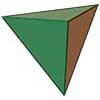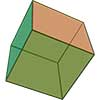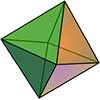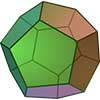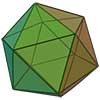Net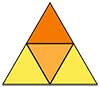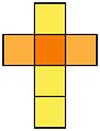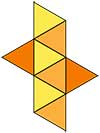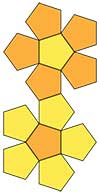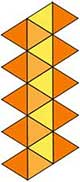Faces 4 6 8 12 20 Face type equilateral triangle square equilateral triangle equilateral pentagon equilateral triangle Duality tetrahedron octahedron hexahedron icosahedron dodecahedron Edges 6 12 12 30 30 Vertices 4 8 6 20 12 Edges from vertex 3 3 4 3 5 Number of diagonals 0 4 3 100 36 Dihedral angle ≈70°31'43,61'' 90° ≈109°28'16,39'' ≈116°33'55,84'' ≈138°11'22,87'' Surface area $\sqrt{3}{a}^{2}$ $6{a}^{2}$ $2\sqrt{3}{a}^{2}$ $3\sqrt{25+10\sqrt{5}}\phantom{\rule{thickmathspace}{0ex}}{a}^{2}$ $5\sqrt{3}{a}^{2}$ Volume $\frac{\sqrt{2}{a}^{3}}{12}$ ${a}^{3}$ $\frac{\sqrt{2}{a}^{3}}{3}$ $\frac{\left(15+7\sqrt{5}\right){a}^{3}}{4}$ $\frac{\left(15+5\sqrt{5}\right){a}^{3}}{12}$ Circumradiu $\frac{\sqrt{6}a}{4}$ $\frac{\sqrt{3}a}{2}$ $\frac{\sqrt{3}a}{2}$ $\frac{\sqrt{3}\left(1+\sqrt{5}\right)a}{4}$ $\frac{\sqrt{10+2\sqrt{5}}\phantom{\rule{thickmathspace}{0ex}}a}{4}$ Inradiu $\frac{\sqrt{6}a}{12}$ $\frac{a}{2}$ $\frac{\sqrt{6}a}{6}$ $\frac{1}{2}\sqrt{\frac{25+11\sqrt{5}}{10}}\phantom{\rule{thickmathspace}{0ex}}a$ $\frac{\sqrt{42+18\sqrt{5}}}{12}\phantom{\rule{thickmathspace}{0ex}}a$ Midradius $\frac{\sqrt{2}a}{4}$ $\frac{\sqrt{2}a}{2}$ $\frac{a}{2}$ $\frac{\left(\sqrt{5}+3\right)a}{4}$ $\frac{\left(1+\sqrt{5}\right)a}{4}$